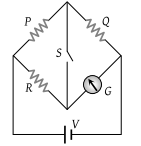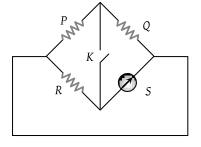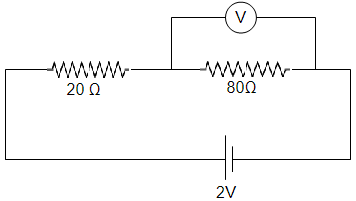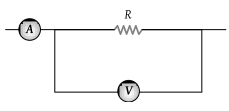Constantan wire is used in making standard resistances because of its :

(1) Specific resistance is low

(2) Density is high

(3) Temperature coefficient of resistance is negligible

(4) Melting point is high

Concept Questions :-

Derivation of Ohm's law
High Yielding Test Series + Question Bank - NEET 2020

Difficulty Level:

A potentiometer consists of a wire of length 4 m and resistance 10 Ω. It is connected to a cell of e.m.f. 2 V. The potential difference per unit length of the wire will be :

(1) 0.5 V/m

(2) 2 V/m

(3) 5 V/m

(4) 10 V/m

Concept Questions :-

Meter bridge and potentiometer
High Yielding Test Series + Question Bank - NEET 2020

Difficulty Level:

In the circuit shown $P\ne R$, the reading of the galvanometer is same with switch S open or closed. Then(1) ${I}_{R}={I}_{G}$

(2) ${I}_{P}={I}_{G}$

(3) ${I}_{Q}={I}_{G}$

(4) ${I}_{Q}={I}_{R}$

Concept Questions :-

Wheatstone bridge
High Yielding Test Series + Question Bank - NEET 2020

Difficulty Level:

In the following Wheatstone bridge $P/Q=R/S$. If key K is closed, then the galvanometer will show deflection :(1) In left side

(2) In right side

(3) No deflection

(4) In either side

Concept Questions :-

Wheatstone bridge
High Yielding Test Series + Question Bank - NEET 2020

Difficulty Level:

In a potentiometer experiment, the galvanometer shows no deflection when a cell is connected across 60 cm of the potentiometer wire. If the cell is shunted by a resistance of 6 Ω, the balance is obtained across 50 cm of the wire. The internal resistance of the cell is

(1) 0.5 Ω

(2) 0.6 Ω

(3) 1.2 Ω

(4) 1.5 Ω

Concept Questions :-

Meter bridge and potentiometer
High Yielding Test Series + Question Bank - NEET 2020

Difficulty Level:

We have a galvanometer of resistance 25 Ω. It is shunted by a 2.5 Ω wire. The part of total current that flows through the galvanometer is given as

(1) $\frac{I}{{I}_{0}}=\frac{1}{11}$

(2) $\frac{I}{{I}_{0}}=\frac{1}{10}$

(3) $\frac{I}{{I}_{0}}=\frac{3}{11}$

(4) $\frac{I}{{I}_{0}}=\frac{4}{11}$

High Yielding Test Series + Question Bank - NEET 2020

Difficulty Level:

In the adjoining circuit, the e.m.f. of the cell is 2 volt and the internal resistance is negligible. The resistance of the voltmeter is 80 ohm. The reading of the voltmeter will be(1) 0.80 volt

(2) 1.60 volt

(3) 1.33 volt

(4) 2.00 volt

High Yielding Test Series + Question Bank - NEET 2020

Difficulty Level:

A voltmeter has resistance of 2000 ohms and it can measure upto 2V. If we want to increase its range to 10 V, then the required resistance in series will be

(1) 2000 Ω

(2) 4000 Ω

(3) 6000 Ω

(4) 8000 Ω

High Yielding Test Series + Question Bank - NEET 2020

Difficulty Level:

The ammeter A reads 2 A and the voltmeter V reads 20 V. the value of resistance R is (Assuming finite resistance's of ammeter and voltmeter)(1) Exactly 10 ohm

(2) Less than 10 ohm

(3) More than 10 ohm

(4) We cannot definitely say

High Yielding Test Series + Question Bank - NEET 2020

Difficulty Level:

The resistance of a galvanometer coil is R. What is the shunt resistance required to convert it into an ammeter of range 4 times

(1) $\frac{R}{5}$

(2) $\frac{R}{4}$

(3) $\frac{R}{3}$

(4) 4 R